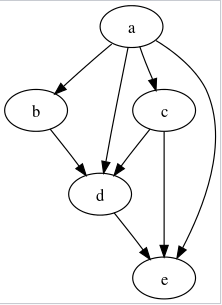New update is available. Click here to update.

# Minimum Number Of Vertices To Reach All Nodes

Last Updated: 31 Mar, 2021
Difficulty: Moderate

## PROBLEM STATEMENT

#### Note :

``````Nodes are numbered from 0 to N - 1.

The graph given is connected.

Print the vertices in sorted order.
``````
##### For Example :
``````The following is an example of DAG i.e a directed graph with no cycles in it.
``````#### In the above graph, we can reach all the vertices from node a.

##### Input Format :
``````The first line contains a single integer ‘T’ denoting the number of test cases. The test cases are as follows.

The first line of each test case contains two integers separated by a single space ‘N’ and ‘M’, where ‘N’  denoting the number of the vertices and ‘M’ denoting the number of edges in the graph.

The next ‘M’ lines of each test case contain two integers ‘X’ and ‘Y’ separated by a single space, here ‘X’ and ’Y’ the vertices connected by a directed edge from ‘X’ to ‘Y’.
``````
##### Output Format :
``````For each test case, print the smallest set of vertices from which all the nodes in the graph are reachable in sorted order.

Print the output of each test case on a new line.
``````
##### Note :
``````You don’t need to print anything; It has already been taken care of. Just implement the given function.
``````
##### Constraints :
``````1 <= T <= 5
2 <= N <= 10^5
1 <= M <= 10^5
0 <= X,Y <= N - 1

Time Limit: 1 sec
``````
##### Sample Input 1 :
``````2
6 5
0 1
0 2
2 5
3 4
4 2
5 4
0 1
2 1
1 4
2 4
``````
##### Sample Output 1 :
``````0 3
0 2 3
``````
##### Explanation of Sample Output 1 :
``````For the first test case,
We can’t cover all the nodes by only one vertex.
From, 0 we can cover 0, 1, 2, 5.
From, 2 we can cover 2, 5.
From, 3 we can cover 2, 3, 4, 5.
From, 5 we can cover 5.

From, 0, 3 we can cover 0, 1, 2, 3, 4, 5.

For the second test case,
From, 0, 2, 3 we can cover the whole graph.
``````

#### Sample Input 2 :

``````2
4 5
0 1
0 2
0 3
1 2
2 3
2 1
1 0
``````
##### Sample Output 2 :
``````0
1
``````## Approach 1

The idea here is that all the vertices with indegree zero should be included in the final ans because these vertices are not reachable from any other nodes. All the other nodes with indegree > 0 can be reached from some other nodes.

The algorithm is as follows:

• Declare an array indegree of size N to store the indegree of each vertex, and for every i set indegree[i] to 0.
• Declare an array ans to store all the nodes using which cover the whole graph.
• Traverse all the edges in the graph,
• Let the current edge be directed from x to y.
• Increment indegree[y] by 1.
• Iterate from vertex = 0 to N - 1,
• If indegree[vertex] is 0,
• Append vertex to ans.
• Return the ans.